# NCERT Miscellaneous Exercise

Question 1: How many words, with or without meaning, each of 2 vowels and 3 consonants can be formed from the letters of the word DAUGHTER?

Answer 1: In the word DAUGHTER, there are 3 vowels namely, A, U, and E, and 5 consonants namely, D, G, H, T, and R.

Number of ways of selecting 2 vowels out of 3 vowels = 3C2 = 3

Number of ways of selecting 3 consonants out of 5 consonants = 5C3 = 10

Therefore, number of combinations of 2 vowels and 3 consonants = 3 × 10 = 30

Each of these 30 combinations of 2 vowels and 3 consonants can be arranged among themselves in 5! ways.

Hence, required number of different words = 30 × 5! = 3600

Question 2: How many words, with or without meaning, can be formed using all the letters of the word EQUATION at a time so that the vowels and consonants occur together?

Answer 2: In the word EQUATION, there are 5 vowels, namely, A, E, I, O, and U, and 3 consonants, namely, Q, T, and N.

Since all the vowels and consonants have to occur together, both (AEIOU) and (QTN) can be assumed as single objects. Then, the permutations of these 2 objects taken all at a time are counted.

This number would be 2P2 = P!

Corresponding to each of these permutations, there are 5! permutations of the five vowels taken all at a time and 3! permutations of the 3 consonants taken all at a time.

Hence, by multiplication principle, required number of words = 2! × 5! × 3! = 1440

Question 3: A committee of 7 has to be formed from 9 boys and 4 girls. In how many ways can this be done when the committee consists of:

(i) exactly 3 girls?                 (ii) atleast 3 girls?                       (iii) atmost 3 girls?

Answer 3:  (i) A committee of 7 has to be formed from 9 boys and 4 girls.

Since exactly 3 girls are to be there in every committee, each committee must consist of (7 – 3) = 4 boys only.

Thus, in this case, required number of ways = 4C3 x 9C4 =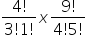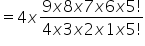= 504

(ii) Since at least 3 girls are to be there in every committee, the committee can consist of (a) 3 girls and 4 boys or (b) 4 girls and 3 boys

3 girls and 4 boys can be selected in 4C3 x 9C4 ways.

4 girls and 3 boys can be selected in 4C4 x 9C3 ways.

Therefore, in this case, required number of ways = 4C3 x 9C4 + 4C4 x 9C3

= 504 + 84 = 588

(iii) Since atmost 3 girls are to be there in every committee, the committee can consist of

(a) 3 girls and 4 boys                  (b) 2 girls and 5 boys

(c) 1 girl and 6 boys                    (d) No girl and 7 boys

3 girls and 4 boys can be selected in 4C3 x 9C4 ways.

2 girls and 5 boys can be selected in 4C2 x 9C5 ways.

1 girl and 6 boys can be selected in 4C1 x 9C6 ways.

No girl and 7 boys can be selected in 4C0 x 9C7 ways.

Therefore, in this case, required number of ways

= 4C3 x 9C4 + 4C2 x 9C5 + 4C1 x 9C6 + 4C0 x 9C7

=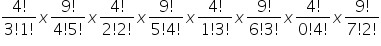= 504 + 756 + 336 + 36

= 1632

Question 4: If the different permutations of all the letter of the word EXAMINATION are listed as in a dictionary, how many words are there in this list before the first word starting with E?

Answer 4: In the given word EXAMINATION, there are 11 letters out of which, A, I, and N appear 2 times and all the other letters appear only once.

The words that will be listed before the words starting with E in a dictionary will be the words that start with A only.

Therefore, to get the number of words starting with A, the letter A is fixed at the extreme left position, and then the remaining 10 letters taken all at a time are rearranged.

Since there are 2 Is and 2 Ns in the remaining 10 letters,

Number of words starting with A =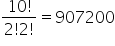Thus, the required numbers of words is 907200.

Question 5: How many 6-digit numbers can be formed from the digits 0, 1, 3, 5, 7 and 9 which are divisible by 10 and no digit is repeated?

Answer 5: A number is divisible by 10 if its units digits is 0.

Therefore, 0 is fixed at the units place.

Therefore, there will be as many ways as there are ways of filling 5 vacant placesin succession by the remaining 5 digits (i.e., 1, 3, 5, 7 and 9).

The 5 vacant places can be filled in 5! ways.

Hence, required number of 6-digit numbers = 5! = 120

Question 6: The English alphabet has 5 vowels and 21 consonants. How many words with two different vowels and 2 different consonants can be formed from the alphabet?

Answer 6: 2 different vowels and 2 different consonants are to be selected from the English alphabet.

Since there are 5 vowels in the English alphabet, number of ways of selecting 2 different

vowels from the alphabet = 5C2 =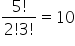Since there are 21 consonants in the English alphabet, number of ways of selecting 2 different consonants from the alphabet = 5C2 =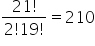Therefore, number of combinations of 2 different vowels and 2 different consonants = 10 × 210 = 2100

Each of these 2100 combinations has 4 letters, which can be arranged among themselves in 4! ways.

Therefore, required number of words = 2100 × 4! = 50400

Question 7: In an examination, a question paper consists of 12 questions divided into two parts i.e., Part I and Part II, containing 5 and 7 questions, respectively. A student is required to attempt 8 questions in all, selecting at least 3 from each part. In how many ways can a student select the questions?

Answer 7: It is given that the question paper consists of 12 questions divided into two parts – Part I and Part II, containing 5 and 7 questions, respectively.

A student has to attempt 8 questions, selecting at least 3 from each part.

This can be done as follows.

(a) 3 questions from part I and 5 questions from part II

(b) 4 questions from part I and 4 questions from part II

(c) 5 questions from part I and 3 questions from part II

3 questions from part I and 5 questions from part II can be selected in 5C3 x 7C5 ways.

4 questions from part I and 4 questions from part II can be selected in 5C4 x 7C4 ways.

5 questions from part I and 3 questions from part II can be selected in 5C5 x 7C3 ways.

Thus, required number of ways of selecting questions

= 5C3 x 7C5 + 5C4 x 7C4 + 5C5 x 7C3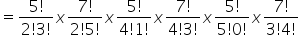= 210 + 175 + 35 = 420

Question 8: Determine the number of 5-card combinations out of a deck of 52 cards if each selection of 5 cards has exactly one king.

Answer 8: From a deck of 52 cards, 5-card combinations have to be made in such a way that in each selection of 5 cards, there is exactly one king.

In a deck of 52 cards, there are 4 kings.

1 king can be selected out of 4 kings in 4C1 ways.

4 cards out of the remaining 48 cards can be selected in 48C4 ways.

Thus, the required number of 5-card combinations is 4C1 x 48C4.

Question 9: It is required to seat 5 men and 4 women in a row so that the women occupy the even places. How many such arrangements are possible?

Answer 9: 4 men and 4 women are to be seated in a row such that the women occupy the even places.

The 5 men can be seated in 5! ways. For each arrangement, the 4 women can be seated only at the cross marked places (so that women occupy the even places).

M x M x M x M x M

Therefore, the women can be seated in 4! ways.

Thus, possible number of arrangements = 4! × 5! = 24 × 120 = 2880

Question 10: From a class of 25 students, 10 are to be chosen for an excursion party. There are 3 students who decide that either all of them will join or none of them will join. In how many ways can the excursion party be chosen?

Answer 10: From the class of 25 students, 10 are to be chosen for an excursion party. Since there are 3 students who decide that either all of them will join or none of them will join, there are two cases.

Case I: All the three students join.

Then, the remaining 7 students can be chosen from the remaining 22 students in 22C7 ways.

Case II: None of the three students join.

Then, 10 students can be chosen from the remaining 22 students in 22C10 ways.

Thus, required number of ways of choosing the excursion party is 22C7 + 22C10.

Question 11: In how many ways can the letters of the word ASSASSINATION be arranged so that all the S’s are together?

Answer 11: In the given word ASSASSINATION, the letter A appears 3 times, S appears 4 times, I appears 2 times, N appears 2 times, and all the other letters appear only once. Since all the words have to be arranged in such a way that all the Ss are together, SSSS is treated as a single object for the time being. This single object together with the remaining 9 objects will account for 10 objects.

These 10 objects in which there are 3 As, 2 Is, and 2 Ns can be arranged in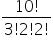ways.

Thus, Required number of ways of arranging the letters of the given word =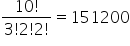Related Keywords